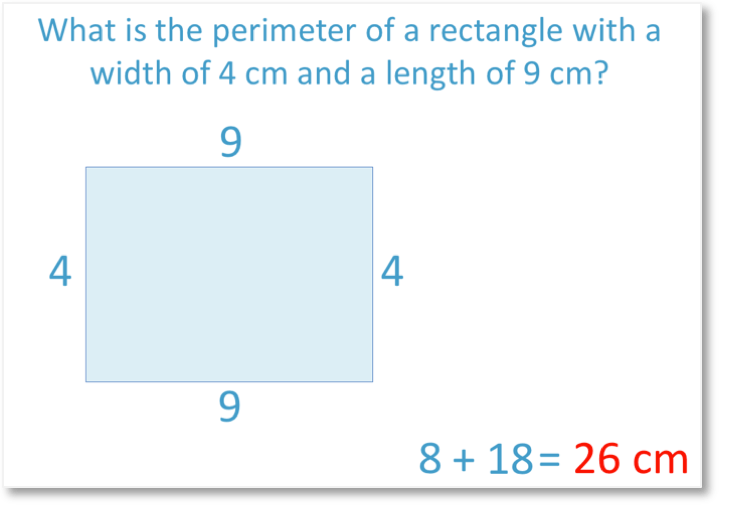# Measuring Millimetres on a Ruler

Measuring Millimetres on a Ruler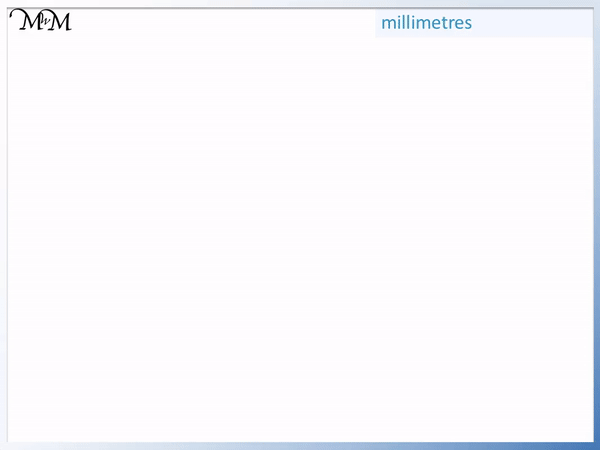• Each of the numbers that are written on a ruler are one centimetre apart from each other.
• In between each of the centimetres, we have ten smaller lines that are one millimetre apart from each other.
• One millimetre is one tenth of a centimetre.
• We can use millimetres to measure things smaller than one centimetre or to measure things more accurately.
• We can write millimetres as ‘mm’ for short.
• We can see that 10 millimetres is the same length as one centimetre.
• We still measure objects starting from the zero line on our ruler.
One millimetre (1 mm) is one tenth of one centimetre (1 cm).

10 mm is the same length as 1 cm.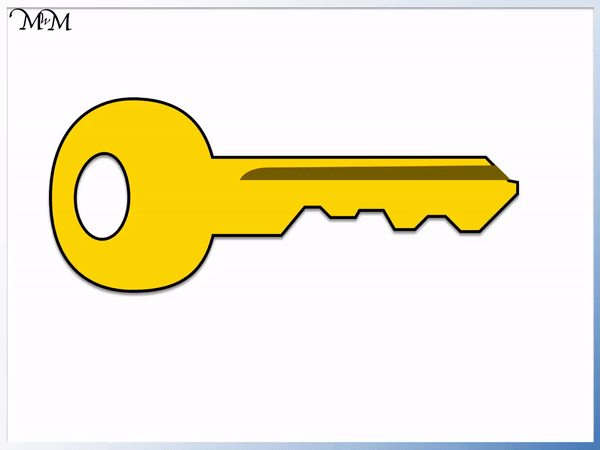• We will measure this key’s length in millimetres.
• Each centimetre is worth 10 mm.
• We can count up in centimetres with each centimetre worth 10 mm.
• To measure the key, we line its left hand side up with the zero line on the ruler.
• The key is larger than 40 mm but less than 50 mm, since the end is between 4 and 5 cm.
• We count on in millimetres from 40 mm until we reach the right end of the key.
• There are 3 small lines after the 4 cm mark and so, the length is 3 mm more than 40 mm.
• The key is 43 mm long.Supporting Lessons#### Measuring Millimetres on a Ruler Worksheets and Answers

Print the sheets below to 100% scale to ensure that the lengths are correct.

# How do you Measure Lengths in Millimetres on a Ruler?

A millimetre is a measurement of length, which is one tenth of a centimetre long. There are 1000 millimetres in one metre and ten millimetres in one centimetre.

Millimetres are often abbreviated to just ‘mm’ for short in the same way that centimetres are abbreviated to ‘cm’.

Each number that is written on a ruler is one centimetre in length.

Between each of the centimetre lines, there are many smaller lines that do not have numbers written on them.

Each of these smallest lines are millimetres and each line is one millimetre in length away from the line next to it.

Below is a ruler with one millimetre (or 1 mm) shown.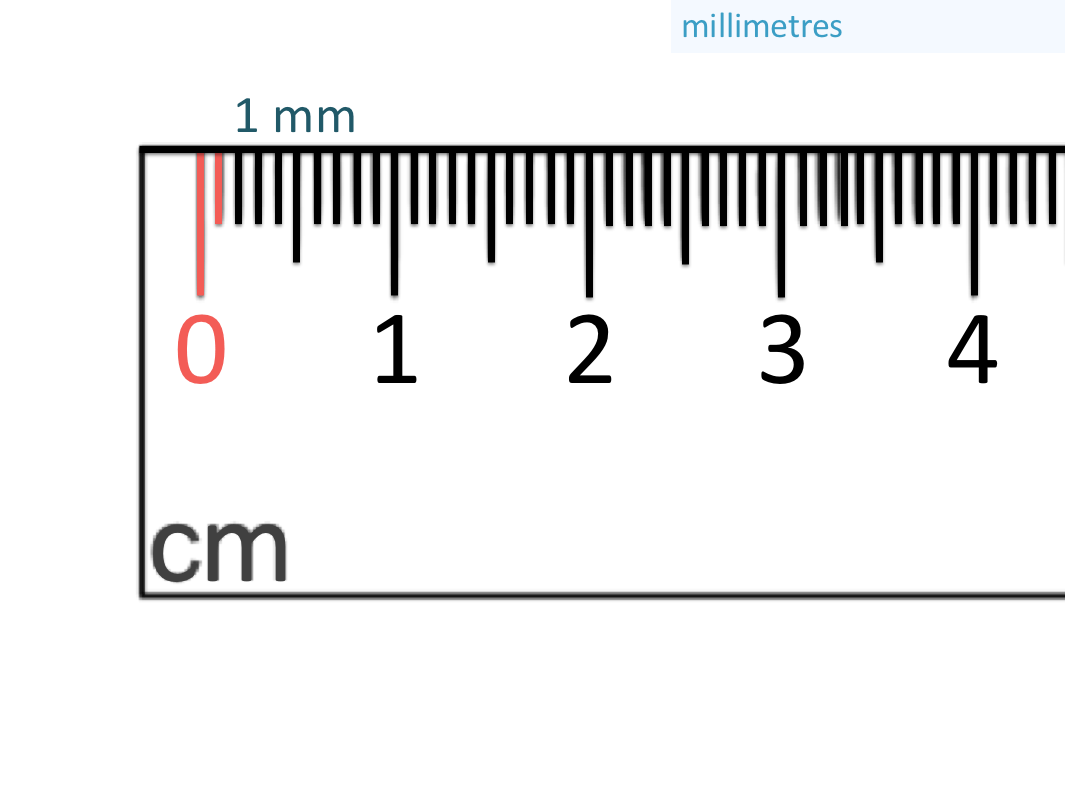Remember that when measuring a length with a ruler, we start from the first line on the left of the ruler, which is the zero line.

The two red lines marked are one millimetre apart.

Since this is the first line we come to after 0, it is 1 mm.

We can continue to count these lines on our ruler.

Here is the second small line and so, this is 2 mm away from zero.We can count further and this line below is 5 mm.This line is slightly longer than the 1 mm, 2 mm, 3mm and 4 mm lines and slightly less than the 0 and 1 cm lines.

5 mm is half way in between 0 and 1 cm.

Therefore 5 mm is half of a centimetre.

The line is slightly longer so that it stands out and we can read half a centimetre more easily.

Once we count past 5 mm, we can count 6 mm, 7 mm, 8 mm, 9 mm and eventually get to 10 mm below.Once we have counted 10 of the smallest lines, we have counted 10 mm.

We can see that we are on the 1 cm line, marked with a ‘1’.

10 mm is the same length as 1 cm.

Every centimetre on a ruler is worth 10 mm.

We will now look at an example of measuring an object in millimetres.

Our first example will be an object with a length that is less than 1 cm long.

Here we have a button and we will measure its width by lining up the leftmost part of the button with the zero line on the ruler as shown below.We count the number of lines that we have until the lines on the ruler line up with the rightmost part of the button.

There are 8 lines and so, the button is 8 mm wide.

We could have counted 8 lines from zero, or we could have looked at the 5 mm line that is in the middle of 0 and 1 cm. From here we have another 3 millimetre lines.

5 mm + 3 mm = 8 mm

Alternatively, we could have seen that the button is almost at the 1 cm line.

The button lines up with a line that is 2 lines away from 1 cm.

1 cm is worth 10 mm and we could have subtracted 2 mm from 10 mm to get to 8 mm.

Below is an example of measuring the side length of a die.

Again we line the left side of the die up with the zero line on the ruler.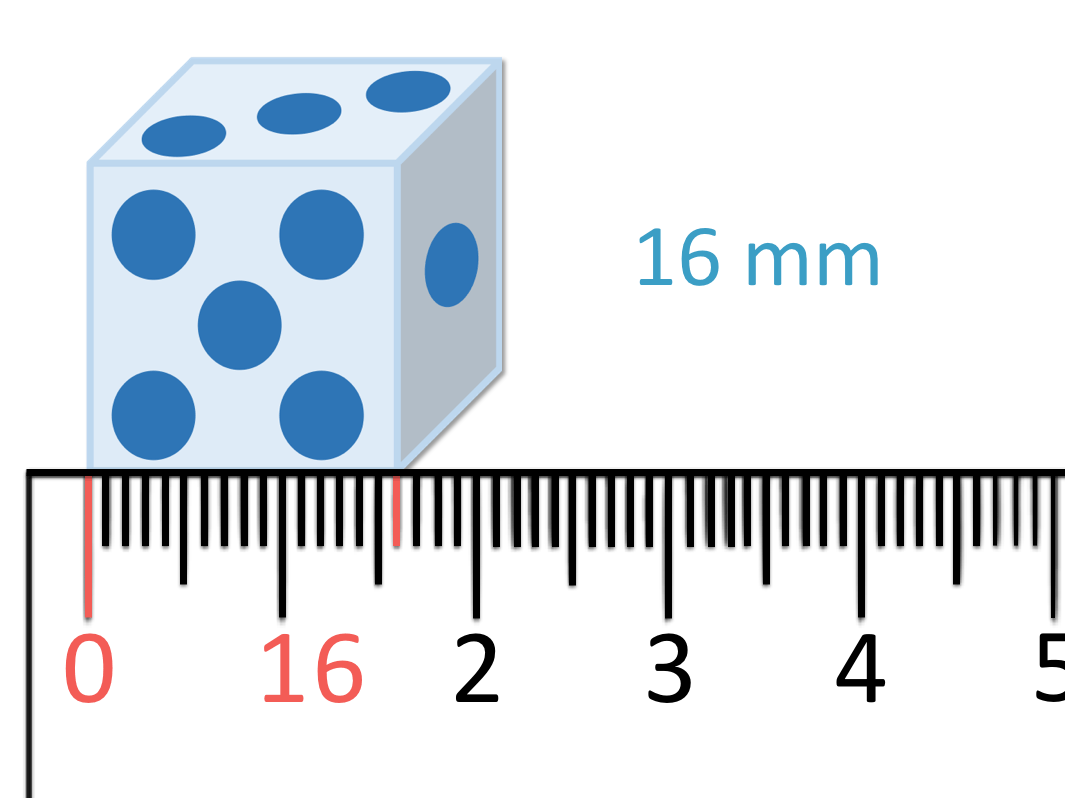The die is 16 mm long.

We could have got this by counting 16 individual lines but this can be quite slow and it is easier to make a mistake in your counting.

The 1 cm mark is worth 10 mm.

We could have counted on 6 more millimetre lines from 10 mm to make 16 mm.

Alternatively, we know that the slightly longer line after the 1 cm mark is another 5 mm.

This is 15 mm, directly in between 1 cm and 2 cm.

The die length is one more line after the 15 mm mark and so it is 16 mm long.

Again we could have also realised that the right side of the die is 4 millimetre lines to the left of the 2 cm mark.

2 cm is worth 20 mm and if we count down 4 places from 20, we get to 16 mm.

We have included this final example to cover another mistake that children might encounter when measuring an image in a book.

The image is showing a 3D die and some children may measure the image all the way to the right hand side, not realising that this is the depth of the die.

It is important to explain that the length we are actually measuring is just the front edge and possibly show them a real die if they are making this mistake.Now try our lesson on How to Find the Perimeter of Rectangles and Squares where we learn how to calculate the perimeter of rectangles by adding their side lengths.